# HSSlive: Plus One & Plus Two Notes & Solutions for Kerala State Board

## AP Board Class 7 Maths Chapter 2 Fractions Decimals and Rational Numbers Ex 1 Textbook Solutions PDF: Download Andhra Pradesh Board STD 7th Maths Chapter 2 Fractions Decimals and Rational Numbers Ex 1 Book AnswersAP Board Class 7 Maths Chapter 2 Fractions Decimals and Rational Numbers Ex 1 Textbook Solutions PDF: Download Andhra Pradesh Board STD 7th Maths Chapter 2 Fractions Decimals and Rational Numbers Ex 1 Book Answers

## Andhra Pradesh State Board Class 7th Maths Chapter 2 Fractions Decimals and Rational Numbers Ex 1 Books Solutions

 Board AP Board Materials Textbook Solutions/Guide Format DOC/PDF Class 7th Subject Maths Chapters Maths Chapter 2 Fractions Decimals and Rational Numbers Ex 1 Provider Hsslive

## How to download Andhra Pradesh Board Class 7th Maths Chapter 2 Fractions Decimals and Rational Numbers Ex 1 Textbook Solutions Answers PDF Online?

2. Click on the Andhra Pradesh Board Class 7th Maths Chapter 2 Fractions Decimals and Rational Numbers Ex 1 Answers.
3. Look for your Andhra Pradesh Board STD 7th Maths Chapter 2 Fractions Decimals and Rational Numbers Ex 1 Textbooks PDF.
4. Now download or read the Andhra Pradesh Board Class 7th Maths Chapter 2 Fractions Decimals and Rational Numbers Ex 1 Textbook Solutions for PDF Free.

## AP Board Class 7th Maths Chapter 2 Fractions Decimals and Rational Numbers Ex 1 Textbooks Solutions with Answer PDF Download

Find below the list of all AP Board Class 7th Maths Chapter 2 Fractions Decimals and Rational Numbers Ex 1 Textbook Solutions for PDF’s for you to download and prepare for the upcoming exams:

Question 1.
Solve the following.
(i) 2 + 34
(ii) 79+13
(iii) 1 – 47
(iv) 223+12
(v) 58−16
(vi) 223+312
Solution: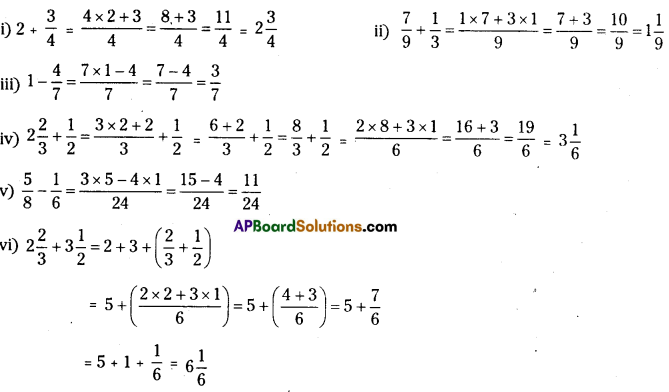Question 2.
Arrange the following in ascending order.
(i) 58,56,12
(ii) 25,13,310
Solution: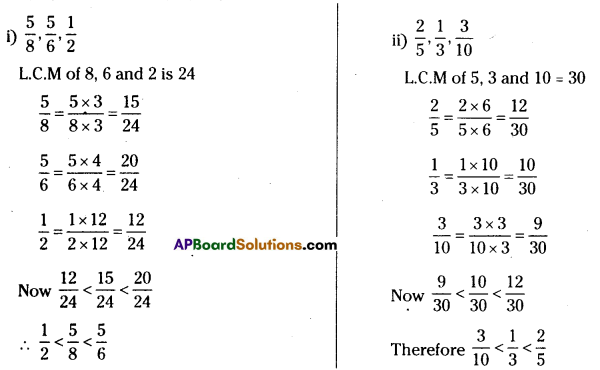Question 3.
Check whether in this square the sum of the numbers in each row and in each column and along the diagonals is the same.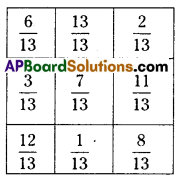Solution: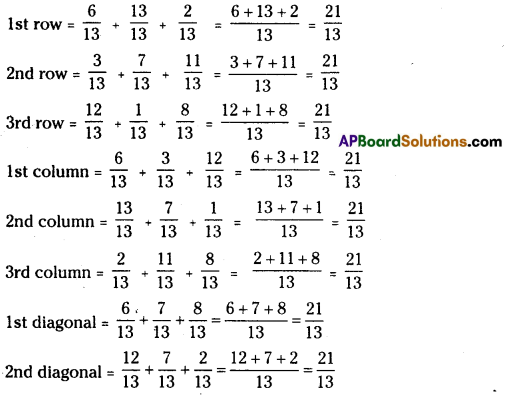Question 4.
A rectangular sheet of paper is 523 cm long and 315 cm wide. Find its perimeter.
Solution:
Length of the rectangular sheet = 523 cm
Breadth/width of the rectangular sheet = 523 cm
Perimeter = 2 x (length + breadth)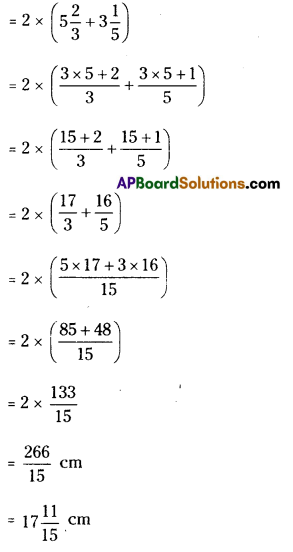Question 5.
The recipe requires 314 cups of flour. Radha has 138 cups of flour. How many more cups of flour does she need?
Solution:
Flour required for the recipe = 314 cups
Flour with Radha = 138 cups
More cups of flour required = 314−138
= 3×4+14−1×8+38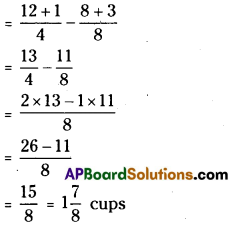Question 6.
Abdul is preparing for his final exam. He has completed 512 part of his course content. Find out how much course content is left?
Solution:
Take content as 1 (i.e., full) Course completed = 512
Course yet to be completed = 1 – 512
= 12×1−512
12−512=712

Question 7.
Find the perimeters of(i) ΔABE (ii) the rectangle BCDE in this figure. Which figure has greater perimetre and by how much?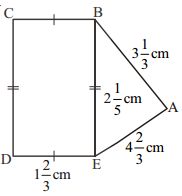Solution:
i) Perimeter of ΔABE = AB + BE + AE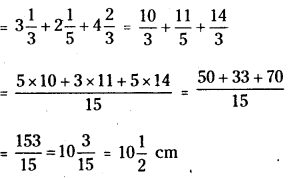ii) Perimeter of BCDE = 2(BE + BC)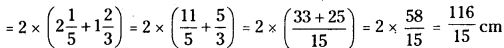As 11615<15315, we conclude that the perimetre of ΔABE > Perimeter of BCDE

## Andhra Pradesh Board Class 7th Maths Chapter 2 Fractions Decimals and Rational Numbers Ex 1 Textbooks for Exam Preparations

Andhra Pradesh Board Class 7th Maths Chapter 2 Fractions Decimals and Rational Numbers Ex 1 Textbook Solutions can be of great help in your Andhra Pradesh Board Class 7th Maths Chapter 2 Fractions Decimals and Rational Numbers Ex 1 exam preparation. The AP Board STD 7th Maths Chapter 2 Fractions Decimals and Rational Numbers Ex 1 Textbooks study material, used with the English medium textbooks, can help you complete the entire Class 7th Maths Chapter 2 Fractions Decimals and Rational Numbers Ex 1 Books State Board syllabus with maximum efficiency.

## FAQs Regarding Andhra Pradesh Board Class 7th Maths Chapter 2 Fractions Decimals and Rational Numbers Ex 1 Textbook Solutions

#### Can we get a Andhra Pradesh State Board Book PDF for all Classes?

Yes you can get Andhra Pradesh Board Text Book PDF for all classes using the links provided in the above article.

## Important Terms

Andhra Pradesh Board Class 7th Maths Chapter 2 Fractions Decimals and Rational Numbers Ex 1, AP Board Class 7th Maths Chapter 2 Fractions Decimals and Rational Numbers Ex 1 Textbooks, Andhra Pradesh State Board Class 7th Maths Chapter 2 Fractions Decimals and Rational Numbers Ex 1, Andhra Pradesh State Board Class 7th Maths Chapter 2 Fractions Decimals and Rational Numbers Ex 1 Textbook solutions, AP Board Class 7th Maths Chapter 2 Fractions Decimals and Rational Numbers Ex 1 Textbooks Solutions, Andhra Pradesh Board STD 7th Maths Chapter 2 Fractions Decimals and Rational Numbers Ex 1, AP Board STD 7th Maths Chapter 2 Fractions Decimals and Rational Numbers Ex 1 Textbooks, Andhra Pradesh State Board STD 7th Maths Chapter 2 Fractions Decimals and Rational Numbers Ex 1, Andhra Pradesh State Board STD 7th Maths Chapter 2 Fractions Decimals and Rational Numbers Ex 1 Textbook solutions, AP Board STD 7th Maths Chapter 2 Fractions Decimals and Rational Numbers Ex 1 Textbooks Solutions,
Share: